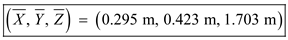# Locate the center of gravity of' the sheet-metal form shown.

Question-AnswerCategory: Engineering MechanicsLocate the center of gravity of' the sheet-metal form shown.

Locate the center of gravity of’ the sheet-metal form shown.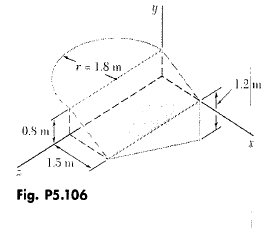Step: 1

Let us draw a diagram of the sheet metal form.Step: 2

Assume that the body is homogeneous so that its center of gravity coincides with the centroid of the area.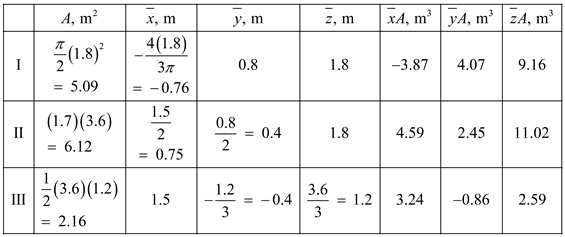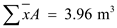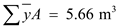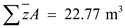Step: 3

We know that,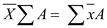. Substitute the known values in the expression.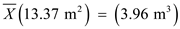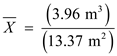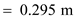Step: 4

We know that,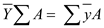Substitute the known values in the expression.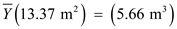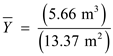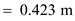Step: 5

We know that,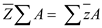Substitute the known values in the expression.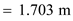The coordinates of the center of gravity